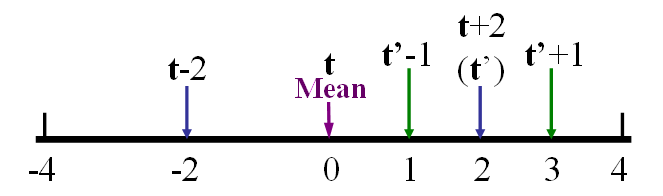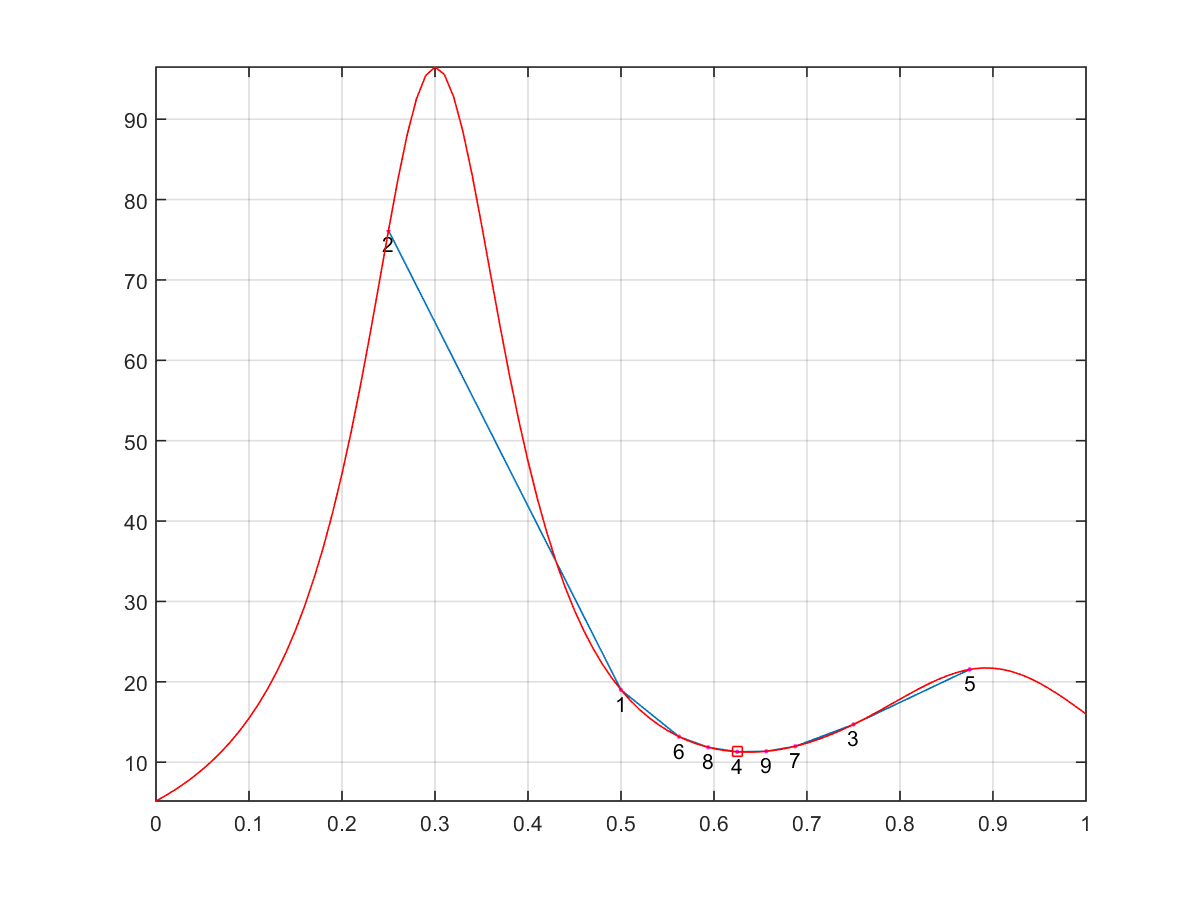## 14-3 Key Transposition (嚙踝蕭嚙踝蕭嚙踝蕭嚙踝蕭嚙踝蕭嚙踝蕭)

[chinese][all]

Since the query input may have a different key than those used in the song database, we need to perform key transposition before comparison.

Depending on the methods for comparison, we have different schemes for key transposition, as follows:

• One-shot method: If we are using linear scaling, we can simple shift the pitch vector to the mean or median of the database entry to guarantee the distance is minimized. This is very efficient and requires less computation.
• Trial-and-error method: If we are using type-1 or type-2 DTW, we will not be able to know the best amount for key transposition in a one-shot manner. As a result, we need to resort to a certain trial-and-error method to find the best pitch shift amount for key transposition.
If we are using trial-and-error methods, we can actually adopt any methods for one-dimensional function optimization for such a purpose. Two commonly used methods are explained next.
• Linear search (exhaustive search):
1. Shift the mean of the input pitch vector t to the same value of the mean of the song in the database. (If the length of the input vector is m, the mean of the database song should be computed based on the same length too.)
2. Add each element of [-2.0, -1.8, -1.6, ... 1.6, 1.8, 2.0] to t and compute the minimum distance between t and r.
• Binary-like search:
1. Shift the mean of the input pitch vector t to the same value of the mean of the song in the database. (If the length of the input vector is m, the mean of the database song should be computed based on the same length too.)
2. Set s = 2.
3. Shift t to each element of [-s, 0, s] and compute the distances to r to find the minimum distance. Shift t according to the minimum distance.
4. Set s = s/2 and go back to the previous step until it converges. See the following schematic plot for the binary-like search:We can use the function binarySearch4min.m in the Utility Toolbox for binary-like search of the minimum of a single-input function. For instance, in the following example, we want to find the minimum of the humps function within [0, 1], and restrict the number of function evaluations to be 9:

Example 1: binarySearch4min01.mobjFunction='humps'; % Objective function optimPrm.searchRange=[0.0, 1.0]; optimPrm.evalCount=9; optimPrm.plotOpt=1; [minPair, allPairs]=binarySearch4min(objFunction, optimPrm); fprintf('minX=%f, minY=%f\n', minPair(1), minPair(2)); x=linspace(optimPrm.searchRange(1), optimPrm.searchRange(2), 101); y=humps(x); line(x, y, 'color', 'r'); grid on set(gca, 'ylim', [min(y), max(y)]);minX=0.625000, minY=11.297297In the above plot, each red point indicates a function evaluation. The number beside each red dot indicates the sequence in function evaluation. The identified minimum is indicated by a red square.

In practice, for different applications, the user can adjust these parameters, including the search range and the number of function evaluation.

Audio Signal Processing and Recognition (音訊處理與辨識)